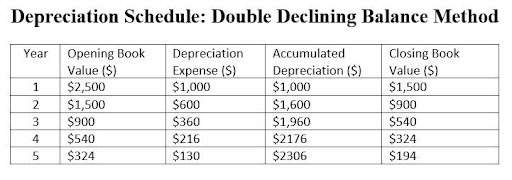## Instead of calculating each ratio individually

Let our ratio tool instantly calculate your ratios. Input your financial data once and get multiple results.

## Depreciation Schedule (Double Declining Balance method)

Double Declining Balance method = (2 x Straight Line Depreciation Rate) x Opening Book Value

### INTERPRETATION

The double declining balance is an accelerated method for recording larger depreciation expenses during the earlier years of an asset’s useful life and then recording smaller depreciation during its later years. In summary, the method involves depreciating certain assets at twice the rate outlined under straight-line depreciation.

Business owners, managers, and other interested parties use it to record the depreciation of technology products that rapidly become obsolete.

Note: expanded calculation
Divide “100%” by the number of years in the asset’s useful life; this is your straight-line depreciation rate. Then, multiply that number by 2, which is your Double-Declining Depreciation Rate. Then multiply by the opening book value.

### EXAMPLE

M&M owner purchased a new company laptop, with a purchase price of \$2500. The useful life of 5 years. Because this laptop will be obsolete at the end of the 5 years, M&M uses the double declining balance method to depreciate the laptop.

Description of asset: Laptop
Year (start): 20XX
Cost of asset: \$2,500
Residual value (salvage value): N/A
Useful Life: 5 years
Depreciation Rate: 20%
Depreciable Cost: N/A

### SEE: Depreciation Schedule - Double Declining Balance Method Diagram

Straight Line Depreciation Rate = 100% / useful life
Depreciation Rate = 100% /5 years = 20%

Depreciation Expense = (2 x Straight Line Depreciation Rate) x Opening Book Value
Depreciation Expense = (2 x 20%) x \$2,500 = \$1,000

Accumulated Depreciation = Depreciation Expense + Accumulated Depreciation
Accumulated Depreciation (year 2) =\$1,000 + \$600 = \$1,600 in year 2

Closing Book Value = Opening Book Value – Depreciation Expense
Closing Book Value = \$2,500 – \$1,000 = \$1,500

### BENCHMARK: ROT

Companies use the double declining balance method for assets that lose most of their value early on or become obsolete more quickly.Depreciation Schedule (Double Declining Balance method) :

### ABBREVIATION KEY:

ROT: Rule of thumb
HA: Historical Average (organization’s historical average)
PG: Peer Group average
EB: Economic Benchmark

DISCLAIMER: The interactive calculators on this site are self-help tools intended to help you visualize and explore your financial information. They are not intended to replace the advice of a qualified professional. Because each business is different, we can not guarantee accuracy.